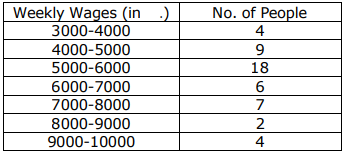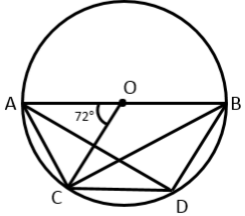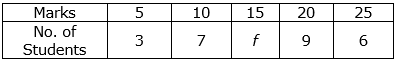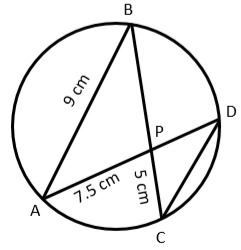ICSE Class 10 Mathematics Previous Year Question Paper 2020

Mathematics is one of the crucial and most scoring subjects in ICSE Class 10TopperLearning presents ICSE Class 10 Mathematics study materials, enabling students to score well in the board examination. The ICSE Class 10 Maths syllabus is diverse and includes challenging concepts like Value Added Tax, Ratio and Proportion, Quadric Equations, and others that require effective study materials.

Our study materials for ICSE Class 10 consist of video lessons, question banks, sample papers, revision notes and past year papers, which improve the quality of learning. The resources also include solutions of top textbooks like Selina and RS Agarwal. Students also refer to the NCERT textbooks as a reference tool.

The problem-solving videos are unique as qualified and experienced experts carefully draft them with an immense research on the chapters' fundamental concepts and their problem sets.

The ICSE Class 10 maths notes are prepared chapter-wise and final revision tools during exams. Students use the UnDoubt section to clarify their academic doubts.

Students prefer to generate practice tests based on multiple-choice questions in the website's ICSE Class 10 Tests section.

To access all these resources and many more, tune in to the TopperLearning website choosing a plan from your ICSE Class 10 Plan section.

SECTION A (40 Marks)
Attempt all questions from this Section.

Question 1

1. Solve the following Quadratic Equation:                                                                                                   
x2 - 7x + 3 = 0
2. GivenIf A2 = 3l, where l is the identify matrix of order 2, find x and y.
3. Using ruler and compass construct a triangle ABC where AB = 3cm, BC = 4 and ∠ABC = 90°. Hence construct a circle circumscribing the triangle ABC. Measure and write down the radius of the circle.                                        

Question 2

1. Use factor theorem to factorise 6x3 + 17x2 + 4x – 12 completely.                                                             

2. Solve the following in equation and represent the solution set on the number line.3. Draw a histogram for the given data, using a graph paper:Estimate the mode from the graph.

Question 3

1. In the figure given below, O is the centre of the circle and AB is a diameter.
If AC = BD and ∠AOC = 72°, Find:                                                                                                         
i. ∠ABC
iii. ∠ABD2. Prove that:3. In what ratio is the line joining P(5, 3) and Q(-5, 3) divided by the y-axis? Also find the coordinates of the point of intersection.                                                                                                                                        

Question 4

1. A solid spherical ball of radius 6 cm is melted and recast into 64 identical spherical marbles. Find the radius of each marble.                                                                                                                                                  

2. Each of the letters of the word ‘AUTHORIZES’ is written on identical circular discsand put in a bag. They are well shuffled. If a disc is drawn at random from the bag, what is the probability that the letter is:                                      
i. A vowel
ii.One of the first 9 letters of the English alphabet which appears in the given word
iii.One of the last 9 letters of the English alphabet which appears in the given word?

3. Mr. Bedi visits the market and buys the following articles:                                                                            
Medicines costing Rs. 950, GST @5%
A pair of shoes costing Rs. 3000,GST @18%A Laptop bag costing Rs. 1000 with a discount of 30% GST @18%
1. Calculate the total amount of GST paid.
2. The total bill amount including GST paid by Mr. Bedi.

SECTION B (40 Marks)
Attempt any four questions from this Section.

Question 5

1. A company with 500 shares of nominal value Rs. 120 declares an annual dividend of 15% Calculate:               
i. the total amount of dividend paid by the company.
ii. annual income of Mr. Sharma who holds 80 shares of the company. If the return percent of Mr. Sharma from his shares is 10%, find the market value of each share.

2. The mean of the following data is 16. Calculate the value of f.1. The 4th, 6th and the last term of a geometric progression are 10, 40 and 640 respectively. If the common ratio is positive, find the first term, common ratio and the number of terms of the series.                                                            

Question 6

1.Find A2 - 2AB + B2.

2. In the given figure AB = 9 cm, PA = 7.5 cm and PC = 5 cm. Chords AD and BC intersect at P.                                 
i. Prove that ΔPAB ~ ΔPCD
ii. Find the length of CD.
iii. Find area of ΔPAB : area of ΔPCD3. From the top of a cliff, the angle of depression of the top and bottom of a tower are observed to be 45° and 60° respectively. If the height of the tower is 20 m.                                                                                                    
Find:
1. the height of the cliff
2. the distance between the cliff and the tower.

Question 7

1. Find the value of ‘p’ if the lines, 5x – 3y + 2 = 0 and 6x – py +7 = 0 are perpendicular to each other. Hence find the equation of a line passing through (–2, –1) and parallel to 6x – py + 7 = 0.                                                           

2. Using properties of proportion find x : y, given:3. In the given figure TP and TQ are two tangents to the circle with centre O, touching at A and C respectively. If ∠BCQ =55° and ∠BAP=60°, find:                                                                                                                                          
1. ∠OBA and ∠OBC
2. ∠AOC
3. ∠ATC

Question 8

1. What must be added to the polynomial 2x3 – 3x2 – 8x, so that it leaves a remainder 10 when divided by 2x+1?    

2. Mr. Sonu has a recurring deposit account and deposits Rs. 750 per month for 2 years. If he gets Rs. 19125 at the time of maturity, find the rate of interest.                                                                                                                     

3. Use graph paper for this question.                                                                                                                    
Take 1 cm = 1 unit on both x and y axes.
1. Plot the following points on your graph sheets:
2. A(–4, 0), B(–3, 2), C(0, 4), D(4, 1) and E(7, 3)
3. Reflect the points B, C, D and E on the x-axis and name them as B’, C’, D’ and E’ respectively.
4. Join the points A, B, C, D, E, E’, D’, C’, B’ and A in order.
5.  iv. Name the closed figure formed.

Question 9

1. 40 student enter for a game of shot – put competition. The distance thrown (in metres) is recorded below:Use a graph paper to draw an ogive for the above distribution.
Use a scale of 2 cm =1 m on one axis and 2 cm = 5 students on the other axis. Hence using your graph find: i. the median ii. Upper Quartile iii. Number of student who cover a distance which is above 1 16 m 2

2.Question 10

1. If the 6th term of an A. P. is equal to four times its first term and the sum of first six terms is 75, find the first term and the common difference.                                                                                                                                     

2. The difference of two natural numbers is 7 and their product is 450. Find the numbers.                                          

3. Use ruler and compass for this question. Construct a circle of radius 4.5 cm. Draw a chord, AB = 6cm.                    
i. Find the locus of points equidistant from A and B. Mark the point where it meets the circle as D.
ii. Join AD and find the locus of points which are equidistant from AD and AB. Mark the point where it meets the circle as C.
iii. Join BC and CD. Measure and write down the length of side CD of the quadrilateral ABCD.

Question 11

1. A model of a high rise building is made to a scale of 1: 50.

i. If the height of the model is 0.8 m, find the height of the actual building.
ii. If the floor area of a flat in the building is 20 m2, find the floor area of that in the model.                                    

2. From a solid wooden cylinder of height 28 cm and diameter 6 cm, two conical cavities are hollowed out. The diameters of the cones are also of 6 cm and height 10.5 cm.Why to choose our study materials for ICSE Class 10 Mathematics?

• Designed in simple and comprehensive language
• Contain 285 video lessons, 37 revision notes, 3709+ questions in bank, set of 6 sample papers and 9 past year papers
• Prepared by subject experts
• Revised from time to time
• Useful for doing quick revision